# Resonance Notes | Study Electrical Engineering SSC JE (Technical) - Electrical Engineering (EE)

## Document Description: Resonance for Electrical Engineering (EE) 2022 is part of Electrical Engineering SSC JE (Technical) preparation. The notes and questions for Resonance have been prepared according to the Electrical Engineering (EE) exam syllabus. Information about Resonance covers topics like and Resonance Example, for Electrical Engineering (EE) 2022 Exam. Find important definitions, questions, notes, meanings, examples, exercises and tests below for Resonance.

Introduction of Resonance in English is available as part of our Electrical Engineering SSC JE (Technical) for Electrical Engineering (EE) & Resonance in Hindi for Electrical Engineering SSC JE (Technical) course. Download more important topics related with notes, lectures and mock test series for Electrical Engineering (EE) Exam by signing up for free. Electrical Engineering (EE): Resonance Notes | Study Electrical Engineering SSC JE (Technical) - Electrical Engineering (EE)
 1 Crore+ students have signed up on EduRev. Have you?

Resonance

It is the condition when the voltage across a circuit becomes in phase with the current supplied to the circuit.
At resonance, the circuit behaves like a resistive circuit.
Power factor of the circuit at resonance becomes = "1"
The resonance may be classified into two groups

1. Series resonnat circuit

2. Parallel resonant circuit.

Series Resonance (RLC series circuit)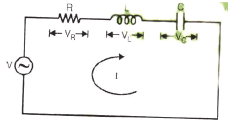The total impedance of series n/w is given by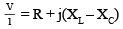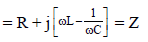where

XL = ωL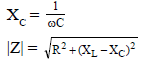At resonance

lm{z} = 0
X– XC = 0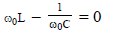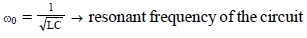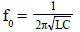where f0 is the frequency of resonance in Hertz
At resonance, the current is I0 = V/R

Variation in Z with respect to 'ω'

Note : For ω < ω0 series RLC behaves like RC capacitive circuit.

ω > ω0 , behaves like RL inductive circuit

ω = ω0 , behaves like resistive circuit.

• Impedance of series RLC circuit is minimum at res onance frequ ency ω = ω0  at resonant frequency Z = R
• Current in series RLC circuit is maximum at resonance frequency

Selectivity and Bandwidth : At frequency of resonance, the impedance of a series RLC circuit is minimum. Hence the current is maximum. As the frequency of the applied voltage deviates on either side of the series resonant frequency, the impedance increases and the current falls. Figure shows the variation of current I with frequency for small values of R. Thus, a series RLC circuit possesses frequency selectivity. The frequencies f1 and f2 at which the current I falls to (1/√2) times its maximum values I0( = V/R) are called halfpower frequencies of 3 - dB frequencies. The bandwidth (f2-f1) is called the halfpower bandwidth or 3-dB bandwidth or simply bandwidth (BW) of the circuit.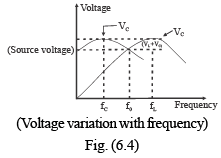Selectivity of a resonant circuit is defined as the ratio of resonant frequency to the BW. Thus,

Selectivity =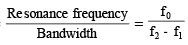Q-factor :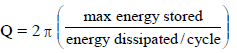• Q of an inductor L with internal resistor R = ωL/R
• Q of a capacitor C with effective internal resistor R = 1/ωCR
• Q of a leaky capacitor which is represented by a capacitor C with a high resistance RP in shunt = ωCRP.

Selectivity increases with decreasing bandwidth For series Resonant circuit at ω = ω0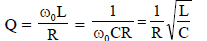For series RLC circuit

BW = ( w2 – w1) =  R/L

Parallel RLC resonnace circuit: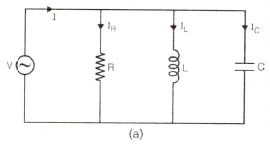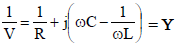At resonance lm (Y) = 0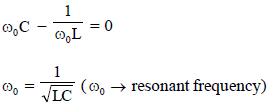at resonance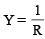Z = R
Properties of Second-Order parallel RLC

Resonant Circuit : A circuit consisting of a parallel connection of a resistor R, an inductor L, and a capacitor C is called a second - order parallel resonant circuit.

The important proporties of such a circuit are as follows:

• The resonant frequency is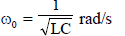• Below resonance, the circuit acts like an RL circuit. 
• Above resonance, the circuit acts like an RC circuit. 
• If the conductance is zero (the resistnace is infinite) ate resonance the circuit acts like an open circuit. 
• The bandwidth is 1/RC. 
• The quality factor QOP is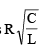Some Conclusions 

• Y = G + j(B– BC)

at resonant frequency Ymin = G

or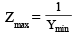• Current will be minimum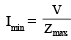• Power factor will be unity i.e. cos ø = 1.
• In parallel resonance net reactive current is equal to zero
• Under resonance condition current flowing through inductor or capacitor is greater than total current.
• This phenomenon is called as current magnification.
• Parallel resonant circuit is also called as antiresonant circuit.

Case-2:

Consider the circuit shown in figure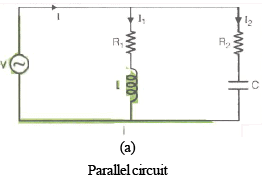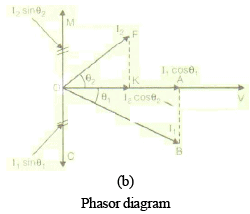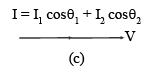Equivalent phasor diagram Fig. (b)

at resonance I1 sinθ1 = I2 sinθ2
In figure (b),

OA = I1 cosθ1

OC = AB = I1 sinθ1

OK = I2 cosθ2

OM = FK = I2 sinθ2
Corresponding to figure (a)

Y = (G1 + G2) + j(BC – BL)
At resonance, BC = BL
Y = G1 + G2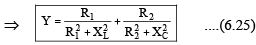Current I = VY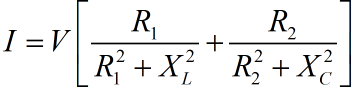Resonant frequency is given by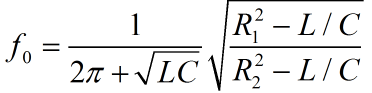Case-3: 

This is a very important case. Observe the tank circuit shown in figure (6.7).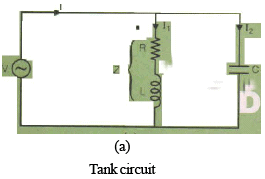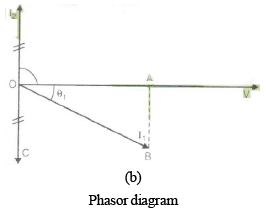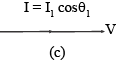Equivalent phasor diagram Fig. (6.7)

In figure 6.7(b),

OA = I1 cosθ

OC = AB = I1 sinθ1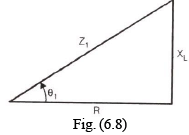and    I1 sinθ1 = I2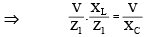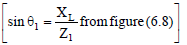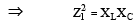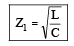Now at resonance, I = I1 cosθ1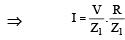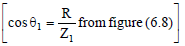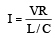[From equation (6.28)]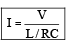....(6.29)
Here L/RC is defined as dynamic impedance of tank circuit i.e.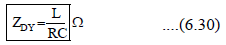And resonance frequency for tank circuit is given by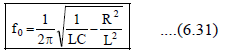Q.factor for Parallel Resonance Circuit 

• Q-factor for parallel resonant circuit is defined as the ratio of current flowing through inductor (or capacitor) to total current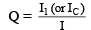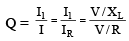⇒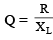⇒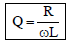.   ..(6.32)

put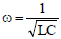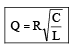...(6.33)

• In another way Q-factor can be obtained as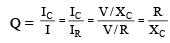⇒ Q = RωC           ...(6.34)

Q-factor of Tank Circuit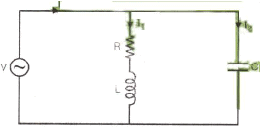Tank circuit Fig.(6.9) 

• Q-factor is given by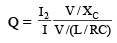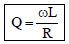...(6.35)

Anti-resonance Curve 

• Anti-resonance curve is shown in figure (6.10)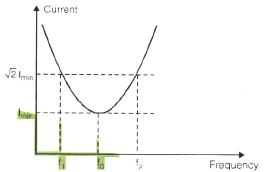Fig. (6.10)

The document Resonance Notes | Study Electrical Engineering SSC JE (Technical) - Electrical Engineering (EE) is a part of the Electrical Engineering (EE) Course Electrical Engineering SSC JE (Technical).
All you need of Electrical Engineering (EE) at this link: Electrical Engineering (EE)

## Electrical Engineering SSC JE (Technical)

2 videos|65 docs|37 tests
 Use Code STAYHOME200 and get INR 200 additional OFF

## Electrical Engineering SSC JE (Technical)

2 videos|65 docs|37 tests

Track your progress, build streaks, highlight & save important lessons and more!

,

,

,

,

,

,

,

,

,

,

,

,

,

,

,

,

,

,

,

,

,

;